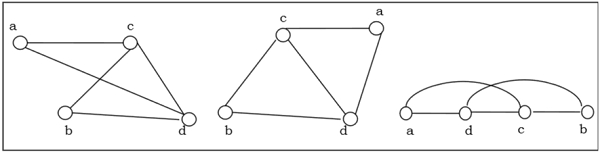# Isomorphism and Homeomorphism of graphs

## Isomorphism

If two graphs G and H contain the same number of vertices connected in the same way, they are called isomorphic graphs (denoted by G ≅ H).

It is easier to check non-isomorphism than isomorphism. If any of these following conditions occurs, then two graphs are non-isomorphic −

• The number of connected components are different
• Vertex-set cardinalities are different
• Edge-set cardinalities are different
• Degree sequences are different

## Example

The following graphs are isomorphic −## Homomorphism

A homomorphism from a graph G to a graph H is a mapping (May not be a bijective mapping) h: G → H such that − (x, y) ∈ E(G) → (h(x), h(y)) ∈ E(H). It maps adjacent vertices of graph G to the adjacent vertices of the graph H.

## Properties of Homomorphisms

• A homomorphism is an isomorphism if it is a bijective mapping.

• Homomorphism always preserves edges and connectedness of a graph.

• The compositions of homomorphisms are also homomorphisms.

• To find out if there exists any homomorphic graph of another graph is a NPcomplete problem.